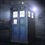# What is the fault here?

Find the number of ordered triplets of integers $x,y,z\ \in\ Z^+$ such that the L.C.M of $x,y,z=2^3\times 3^3$? My approach:

Since we are talking about the lease common multiple we can safely assume that all $x,y,z$ are of the form $x=2^a \times 3^d,y=2^b \times 3^e,z=2^c \times 3^f$ where $a,b,c,d,e,f$ are non negative integers.Now we have that $max(a,b,c)=3,max(d,e,f)=3$,so at least one of $(a,b,c)=3$ and same with $(d,e,f)$,now there are three ways of choosing that one,and after that is done the two left can be $(0,1,2,3$) hence $4$ choices,so the answer should be $(3 \times 4^2)^2=(48)^2=2304$,but it isnt,can somebody point out the mistake please.5 years, 6 months ago

This discussion board is a place to discuss our Daily Challenges and the math and science related to those challenges. Explanations are more than just a solution — they should explain the steps and thinking strategies that you used to obtain the solution. Comments should further the discussion of math and science.

When posting on Brilliant:

• Use the emojis to react to an explanation, whether you're congratulating a job well done , or just really confused .
• Ask specific questions about the challenge or the steps in somebody's explanation. Well-posed questions can add a lot to the discussion, but posting "I don't understand!" doesn't help anyone.
• Try to contribute something new to the discussion, whether it is an extension, generalization or other idea related to the challenge.

MarkdownAppears as
*italics* or _italics_ italics
**bold** or __bold__ bold
- bulleted- list
• bulleted
• list
1. numbered2. list
1. numbered
2. list
Note: you must add a full line of space before and after lists for them to show up correctly
paragraph 1paragraph 2

paragraph 1

paragraph 2

[example link](https://brilliant.org)example link
> This is a quote
This is a quote
    # I indented these lines
# 4 spaces, and now they show
# up as a code block.

print "hello world"
# I indented these lines
# 4 spaces, and now they show
# up as a code block.

print "hello world"
MathAppears as
Remember to wrap math in $$ ... $$ or $ ... $ to ensure proper formatting.
2 \times 3 $2 \times 3$
2^{34} $2^{34}$
a_{i-1} $a_{i-1}$
\frac{2}{3} $\frac{2}{3}$
\sqrt{2} $\sqrt{2}$
\sum_{i=1}^3 $\sum_{i=1}^3$
\sin \theta $\sin \theta$
\boxed{123} $\boxed{123}$

Sort by:

Siddhartha is correct; your method involves some double-counting. I looked at this case by case: let $j$ be the number of elements of $(a,b,c)$ that equal $3$ and $k$ be the number of elements of $(d,e,f)$ that equal $3$, with both $j$ and $k$ ranging from $1$ to $3$. There are then $3*3 = 9$ case pairs $(j,k)$ to analyze.

For example, for $(j,k) = (1,1)$ we can choose one of $(a,b,c)$ in $3$ ways, one of $(d,e,f)$ in $3$ ways, and the remaining four powers can each be any of $0,1$ or $2.$ This results in a total of $3^{6} = 729$ ordered triples $(x,y,z)$.

With $N(j,k)$ being the number of ordered triples $(x,y,z)$ resulting from particular values of $(j,k)$, I find that

$N(1,1) = 3^{6}, N(1,2) = N(2,1) = 3^{5}, N(2,2) = 3^{4}, N(1,3) = N(3,1) = 3^{3}, N(2,3) = N(3,2) = 3^{2}, N(3,3) = 1.$

These values sum to a total of $1369$ ordered triples $(x,y,z)$.

- 5 years, 6 months ago

A simpler solution is the square of the number of ways to choose (a,b,c) from (0,1,2,3) minus the number of ways to choose (a,b,c) from (0,1,2). This is $(4^3 - 3^3)^2 = (37)^2 = 1369$

- 5 years, 6 months ago

well can u please explain the logic behind your answer? i mean how did you get that expression

- 5 years, 6 months ago

Max(a,b,c) = 3. Therefore each of $(a,b,c)$ can take values from $(0,1,2,3)$. This is $4^3$.

Now, we subtract cases where none of $(a,b,c)$ are $3$.

This is equivalent to the cases where values of $(a,b,c)$ is from $0,1,2$. This is $3^3$.

Therefore the required value is $4^3 - 3^3$.We square this since max(d,e,f) =3 also has $4^3 - 3^3$ cases

- 5 years, 6 months ago

ohh thanks a lot i get it

- 5 years, 6 months ago

Ohk thanx a lot for helping me!

- 5 years, 6 months ago

You're double counting many cases. For example, you count the case (3,3,0) two times.

- 5 years, 6 months ago

Thanx for pointing out my mistake.

- 5 years, 6 months ago

Since we are taking LCM so we can assume x = 2^a 3^d, y = 2^b 3^e, z = 2^c 3^f, where a, b, c, d, e, f, g are non negative integers. As per question max {a, b, c} = 3 and max {d, e, f} = 3. Number of ways in which max {a, b, c} = 3 is 4^3 – 3^3 = 37. Similarly, number of cases for max {d, e, f} = 3 is 37. Hence numbers of required ordered triplets = 37 × 37 = 1369.

- 5 years, 2 months ago